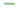# Converting (binary,hex,octal) to decimal numbers in JavaScriptIn the last tutorial, we have learned how to convert decimal to (binary, hexadecimal, octal) numbers. In this tutorial, we are going to learn how to convert binary, hexadecimal and octal to decimal numbers in JavaScript.

## parseInt

In JavaScript, we have the `parseInt()` method by using that we can make conversions.

The parseInt( ) method takes the two arguments, the first argument is `string` and the second argument is `base` by using that it returns the decimal number of a specified base.

Let’s see the examples.

## Converting binary to decimal

To convert a binary to decimal we need to pass base `2` as a second argument to the `parseInt` method.

``````const binaryNumber = "1100";

console.log(parseInt(binaryNumber,2)); // base 2

//output--> 12``````

To convert a hexadecimal to decimal we need to pass base `16` as a second argument to the `parseInt` method.

``````const hex = "c";

console.log(parseInt(hex,16));  //hex base-16

//output --> 12``````

## Converting octal to decimal

To convert a octal to decimal we need to pass base `8` as a second argument to the `parseInt` method.

``````const octal = "14";

console.log(parseInt(octal,8));  //octal base-8

//output --> 12``````

## Top Udemy Courses##### JavaScript - The Complete Guide 2020 (Beginner + Advanced)
45,614 students enrolled
52 hours of video content
View Course##### React - The Complete Guide (incl Hooks, React Router, Redux)
284,472 students enrolled
40 hours of video content
View Course##### Vue JS 2 - The Complete Guide (incl. Vue Router & Vuex)
130,921 students enrolled
21 hours of video content
View Course